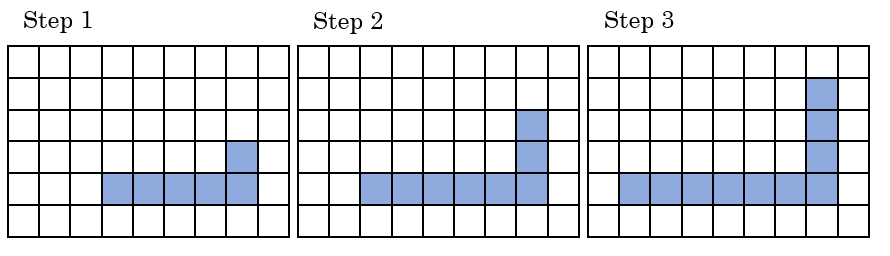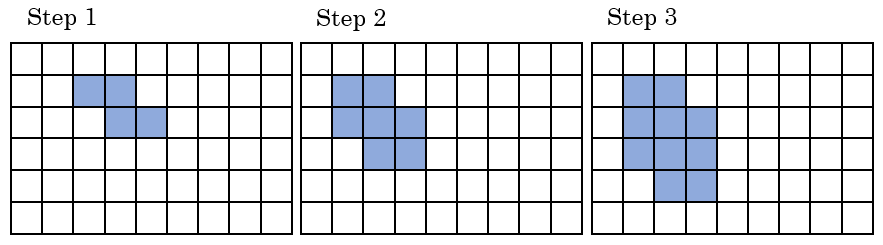# Steps and Squares

In this learning experience, you will represent linear trends in patterns, tables, graphs, and equations with a focus on the change in the number of shaded squares for each step.  After finishing with the worksheet and checking your answer and or Desmos, close Pattern 1:

Use the Worksheet for Pattern 1 and the instructions below to complete this task. Coordinate plane by Desmos.com.

###### Pattern 11. Examine Steps 1, 2, and 3.  Draw Step 0 and Step 4 on the worksheet provided.
2. By how many shaded squares does the pattern grow for each step? Describe the growth pattern in words.
3. Create a table to record the number of shaded squares for each step.  Let $$n$$ represent the step number, and let $$s$$ represent the number of shaded squares.
4. Graph the number of shaded squares for each step.
5. Write an equation to determine the number of shaded squares $$(n)$$ given any step number $$(s)$$.
6. Determine the number of shaded squares in Step 100.### Pattern 2: Use the Worksheet for Pattern 2 and the instructions below to complete this task. Coordinate plane by Desmos.com.

###### Pattern 21. Examine Steps 1, 2, and 3.  Draw Step 0 and Step 4 on the worksheet provided.
2. By how many shaded squares does the pattern grow for each step? Describe the growth pattern in words.
3. Create a table to record the number of shaded squares for each step.  Let $$n$$ represent the step number, and let $$s$$ represent the number of shaded squares.
4. Graph the number of shaded squares for each step.
5. Write an equation to determine the number of shaded squares $$(n)$$ given any step number $$(s)$$.
6. Determine the number of shaded squares in Step 100.

### Pattern 3: Use the Worksheet for Pattern 3 and the instructions below to complete this task. Coordinate plane by Desmos.com.

###### Pattern 31. Examine Steps 1, 2, and 3.  Draw Step 0 and Step 4 on the worksheet provided.
2. By how many shaded squares does the pattern grow for each step? Describe the growth pattern in words.
3. Create a table to record the number of shaded squares for each step.  Let $$n$$ represent the step number, and let $$s$$ represent the number of shaded squares.
4. Graph the number of shaded squares for each step.
5. Write an equation to determine the number of shaded squares $$(n)$$ given any step number $$(s)$$.
6. Determine the number of shaded squares in Step 100.

### Pattern 4: Use the Worksheet for Pattern 4  and the instructions below to complete this task. Coordinate plane by Desmos.com..

###### Pattern 41. Examine Steps 1, 2, and 3.  Draw Step 0 and Step 4 on the worksheet provided.
2. By how many shaded squares does the pattern grow for each step? Describe the growth pattern in words.
3. Create a table to record the number of shaded squares for each step.  Let $$n$$ represent the step number, and let $$s$$ represent the number of shaded squares.
4. Graph the number of shaded squares for each step.
5. Write an equation to determine the number of shaded squares $$(n)$$ given any step number $$(s)$$.
6. Determine the number of shaded squares in Step 100.